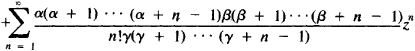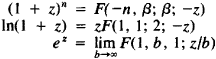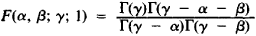# Hypergeometric Series

Also found in: Wikipedia.

## hypergeometric series

[‚hī·pər‚jē·ə′me·trik ′sir·ēz]
(mathematics)
A particular infinite series which in certain cases is a solution to the hypergeometric equation, and having the form:

## Hypergeometric Series

a series of the form

F(α, β; γ; z) = 1The hypergeometric series was studied for the first time by L. Euler in 1778. Expansions of many functions into infinite series are special cases of hypergeometric series. For example,The hypergeometric series has meaning if γ is not equal to zero or a negative integer. It converges at |z| < 1. If, in addition, γ - α - β > 0, then the hypergeometric series also converges for z = 1. In this case, Gauss’ formula is valid:where Γ(z) is the gamma function. An analytic function, defined for |z| < 1 by a hypergeometric series is called a hypergeometric function and plays an important role in the theory of differential equations.

References in periodicals archive ?
of the generalized hypergeometric series (the both sides of this equality are rational functions of [a.
This paper presents a new method for finding identities for hypergeometric series, covering the Appell-Lauricella hypergeometric series, the (Gauss) hypergeometric series, and the generalized hypergeometric series.
We now give the definition of the hypergeometric series which will be used in obtaining some integrals.
They are given as Gaussian hypergeometric series in certain cases where the series is in fact finite
Rahman, Basic Hypergeometric Series, Cambridge University Press, Cambridge, 1990.
0], then the hypergeometric series is a polynomial of degree min{-[a.
Daoust, A note on the convergence of Kampe de Feriet doubles hypergeometric series, Math.
Multiple Gaussian hypergeometric series, ISBN: 0-470-20100-2, Halsted Press [John Wiley & Sons], New York, 1985.
Rahman, Basic Hypergeometric Series, 2nd Edition, (2004), Encyclopedia of Mathematics and Its Applications, 96, Cambridge University Press, Cambridge.
Again Noor  by using hypergeometric series [sub.
In 1880 Paul Emile Appell (1855-1930)  introduced some 2-variable hypergeometric series now called the Appell functions.

Site: Follow: Share:
Open / Close